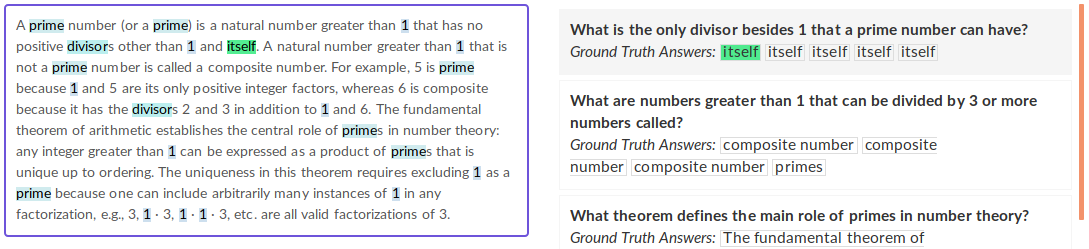## Getting Started

You'll need the following in the root of your repository:

• `sotabench.py` file - contains benchmarking logic; the server will run this on each commit
• `requirements.txt` file - Python dependencies to be installed before running `sotabench.py`
• `sotabench_setup.sh` (optional) - any advanced dependencies or setup, e.g. compilation

You can write whatever you want in your `sotabench.py` file to get model predictions on the SQuAD dataset.

But you will need to record your results for the server, and you'll want to avoid doing things like downloading the dataset on the server. So you should:

• Include an Evaluation object in `sotabench.py` file to record the results.
• Use Caching (optional) - to speed up evaluation by hashing the first batch of predictions.

We explain how to do these various steps below.

## How Do I Initialize an Evaluator?

Add this to your code - before you start batching over the dataset and making predictions:

```from sotabencheval.question_answering import SQuADEvaluator, SQuADVersion

```

If you are reproducing a model from a paper, then you can enter the arXiv ID. If you put in the same model name string as on the SQuAD 1.1 or SQuAD 2.0 leaderboard then you will enable direct comparison with the paper's model. For example:

```from sotabencheval.question_answering import SQuADEvaluator, SQuADVersion

paper_arxiv_id='1907.10529',
```

The above will directly compare with the result of the paper when run on the server.

## Server Data Location

The SQuAD development data is located in the root of your repository on the server at `.data/nlp/squad`. In this folder is contained:

• `dev-v1.1.json` - containing SQuAD v1.1 development dataset
• `dev-v2.0.json` - containing SQuAD v2.0 development dataset

You can use `evaluator.dataset_path: Path` to get a path to the dataset json file. In the example above it resolves to `.data/nlp/squad/dev-v2.0.json` on sotabench server and `./dev-v2.0.json` when run locally. If you want to use a non-standard file name or location when running locally you can override the defaults like this:

```evaluator = SQuADEvaluator(
...,
local_root='mydatasets',
dataset_filename='data.json'
)
```

## How Do I Evaluate Predictions?

The evaluator object has an `.add(answers: Dict[str, str])` method to submit predictions by batch or in full.

For SQuAD the expected input is a dictionary, where keys are question ids and values are text answers. For unanswerable questions the answer should be an empty string. For example:

```{"57296d571d04691400779413": "itself", "5a89117e19b91f001a626f2d": ""}
```

You can do this all at once in a single call to `add()`, but more naturally, you will probably loop over the dataset and call the method for the outputs of each batch. That would look something like this (for a PyTorch example):

```...

paper_arxiv_id="1710.10723",

for i, (input, target) in enumerate(data_loader):
...
output = model(input)
# potentially formatting of the output here to be a dict
```

When you are done, you can get the results locally by running:

```evaluator.get_results()
```

But for the server you want to save the results by running:

```evaluator.save()
```

This method serialises the results and model metadata and stores to the server database.

## How Do I Cache Evaluation?

Sotabench reruns your script on every commit. This is good because it acts like continuous integration in checking for bugs and changes, but can be annoying if the model hasn't changed and evaluation is lengthy.

Fortunately sotabencheval has caching logic that you can use.

The idea is that after the first batch, we hash the model outputs and the current metrics and this tells us if the model is the same given the dataset. You can include hashing within an evaluation loop like follows (in the following example for a PyTorch repository):

```with torch.no_grad():
for i, (input, target) in enumerate(data_loader):
...
output = model(input)
# potentially formatting of the output here to be a list of dicts

if evaluator.cache_exists:
break

evaluator.save()
```

If the hash is the same as in the server, we infer that the model hasn't changed, so we simply return hashed results rather than running the whole evaluation again.

Caching is very useful if you have large models, or a repository that is evaluating multiple models, as it speeds up evaluation significantly.

## A Full sotabench.py Example

Below we show an implementation for a model from the AllenNLP repository. This incorporates all the features explained above: (a) using the SQuAD Evaluator, (b) using custom dataset location when run locally, and (c) the evaluation caching logic.

```from sotabencheval.question_answering import SQuADEvaluator, SQuADVersion

from allennlp.data.iterators import DataIterator
from allennlp.nn.util import move_to_device

model = archive.model
iterator_params = archive.config["iterator"]
iterator_params["batch_size"] = batch_size
data_iterator = DataIterator.from_params(iterator_params)
data_iterator.index_with(model.vocab)

def evaluate(model, dataset, data_iterator, evaluator):
model.eval()
evaluator.reset_time()
for batch in data_iterator(dataset, num_epochs=1, shuffle=False):
batch = move_to_device(batch, 0)
predictions = model(**batch)
if evaluator.cache_exists:
break